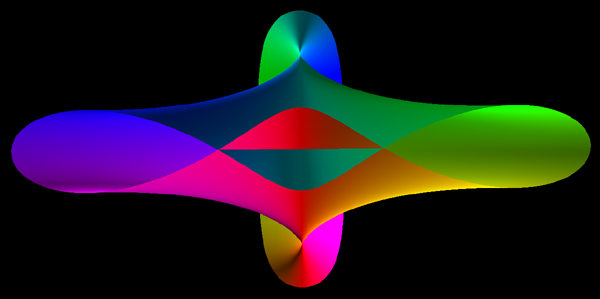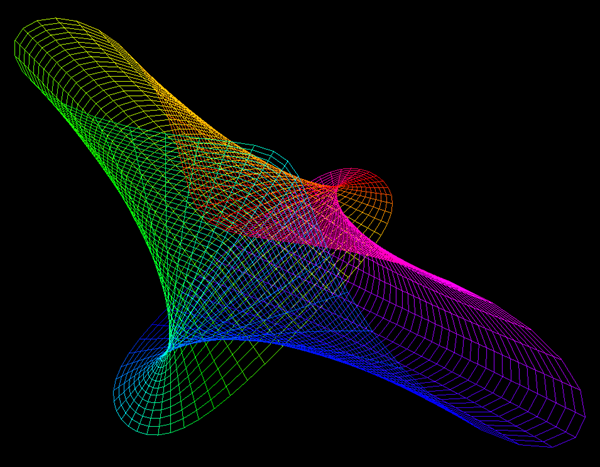# Verrill minimal surface

An Enneper-Weierstrass Minimal Surface
Based on a K3 Elliptical Symmetry

Mathematics by Roger L. Bagula
4 July 2000
Graphics by Paul Bourke

Appeared on stickers for the MAA
(Mathematical Association of America).

Equations

x = -2 r cos(p) + 2 cos(p) / r - 2 r3 cos(3 p) / 3
y = 6 r sin(p) - 2 sin(p) / r - 2 r3 sin(3 p) / 3
z = 4 log(r)
Where 0.5 <= r <= 1 and 0 <= p <= 2 piThe source code creates polygonal facets in the "geom" format. Most of the complexity comes from deriving colours and normals at each vertex, of course this is necessary in order to get a smooth surface with a limited polygonal representation.